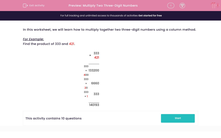# Multiply Two Three-Digit Numbers

In this worksheet, student multiply two three-digit numbers using a column method.Key stage:  KS 2

Curriculum topic:   Maths and Numerical Reasoning

Curriculum subtopic:   Mixed Problems

Difficulty level:#### Worksheet Overview

In this worksheet, we will learn how to multiply together two three-digit numbers using a column method.

For Example:

Find the product of 333 and 421.

 × 333 421 333 × 400 133200 333 × 20 6660 333 × 1 333 140193

### What is EdPlace?

We're your National Curriculum aligned online education content provider helping each child succeed in English, maths and science from year 1 to GCSE. With an EdPlace account you’ll be able to track and measure progress, helping each child achieve their best. We build confidence and attainment by personalising each child’s learning at a level that suits them.

Get started